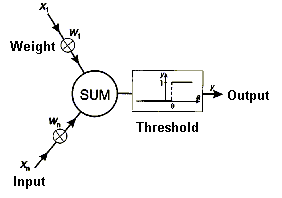# A Simple Artificial Neuron

## <The TLU>The input lines gather in multiple input signals. Each input signal is multiplied by a weight to supply a weighted signal to the summation unit, where the values are summed to supply the input activation to the threshold logic unit. The threshold logic unit compares the input activation to a threshold value. If that value is exceeded, an output of 1 is generated, if not, an output of 0 is generated. The output lines distribute the output signal to multiple destinations.

## Input

Each TLU can have any number of inputs. Each input collects a signal either from outside the network or from other units within the network. Usually the signal is Boolean, only taking the values 1 or 0 (or +1,-1).

A single input corresponds to an afferent synapse on the dendritic tree of a biological neuron, and the signal corresponds to the synapse firing or not firing within a given time period. The number of inputs defines the dimensionality of the state space within which the neuron operates: One dimension for each input, and one for the threshold value. The values of the inputs define a point within that space.

## Weight

The TLU has the same number of weight functions as inputs. There is a one to one relationship between each function and input. The weight function multiplies the input signal by a weight value before returning the result in the summation function.

An inputs' weight value in a simple artificial neuron corresponds to the shape and electro-chemical properties of the paths in the dendritic tree of the biological neuron. In the biological neuron these paths lead from the associated input synapse to the axonal hillock. The weight values define the orientation of the hyperplane that bisects the space defined by the inputs.

## Summation

A single TLU has one summation function. The values of all the weight functions are added together by the summation function before the output is returned by the activation function.

The summation function corresponds to the convergence of the dendritic tree at the soma, and the integration over time of the signals as they arrive at the axonal hillock. The weight and summation functions project the point defined by the values of the inputs along to the threshold axis onto the hyperplane defined by the weight values.

## Threshold

Each artificial neuron has one activation function. In a TLU the activation function is a threshold function. The value of the summation is compared to a threshold value. If the sum is greater than the threshold, the function takes the value 1, otherwise the function takes the value 0.

The activation function corresponds to the collection of an action potential at the axonal hillock. The higher the potential needed to fire a signal down the axon, the higher the "threshold" of the neuron.

 The threshold value defines a hyperplane orthogonal to the threshold axis. This threshold hyperplane can bisect the hyperplane defined by the weight values, so one part will be above the threshold, one below. The threshold function tests the input value projected onto the hyperplane. If the input is projected on the part above the threshold, the activation is 1, if it is projected on the part below the threshold, the activation is 0.## Output

Each TLU can have any number of outputs. Each output takes the value of the activation function and passes it either to a network output or to other units within the network.